Courses

# Income & Cross Elasticity of Demand CA Foundation Notes | EduRev

## Business Economics for CA Foundation

Created by: Sushil Kumar

## CA Foundation : Income & Cross Elasticity of Demand CA Foundation Notes | EduRev

The document Income & Cross Elasticity of Demand CA Foundation Notes | EduRev is a part of the CA Foundation Course Business Economics for CA Foundation.
All you need of CA Foundation at this link: CA Foundation

Income Elasticity of Demand

Income elasticity of demand is the degree of responsiveness of quantity demanded of a good to changes in the income of consumers. In symbolic form,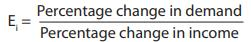This can be given mathematically as follows: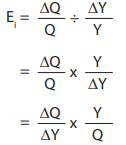Ei = Income elasticity of demand
∆Q = Change in demand
Q = Original demand
Y = Original money income
∆Y = Change in money income

There is a useful relationship between income elasticity for a good and the proportion of income spent on it. The relationship between the two is described in the following three propositions:
1. If the proportion of income spent on a good remains the same as income increases, then income elasticity for the good is equal to one.
2. If the proportion of income spent on a good increase as income increases, then the income elasticity for the good is greater than one.
3. If the proportion of income spent on a good decrease as income rises, then income elasticity for the good is less than one.

Income elasticity of goods reveals a few very important features of demand for the goods in question. If income elasticity is zero, it signifies that the demand for the good is quite unresponsive to changes in income. When income elasticity is greater than zero or positive, then an increase in income leads to an increase in demand for the good. This happens in the case of most of the goods and such goods are called normal goods. For all normal goods, income elasticity is positive. However, the degree of elasticity varies according to the nature of commodities. As against this, goods having negative income elasticity are known as inferior goods and their demand falls as income increases. The reason is that when income increases, consumers choose to consume superior substitutes. Another significant value of income elasticity is that of unity. When income elasticity of demand is equal to one, the proportion of income spent on goods remains the same as consumer’s income increases. This represents a useful dividing line. If the income elasticity for a good is greater than one, it shows that the good bulks larger in consumer’s expenditure as he becomes richer. Such goods are called luxury goods. On the other hand, if the income elasticity is less than one, it shows that the good is either relatively less important in the consumer’s eye or, it is a necessity.

The following examples will make the above concepts clear:
(a) The income of a household rises by 10%, the demand for wheat rises by 5%.
(b) The income of a household rises by 10%, the demand for T.V. rises by 20%.
(c) The incomes of a household rises by 5%, the demand for bajra falls by 2%.
(d) The income of a household rises by 7%, the demand for commodity X rises by 7%.

(e) The income of a household rises by 5%, the demand for buttons does not change at all.

Using formula for income elasticity,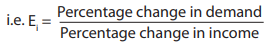we will find income-elasticity for various goods. The results are as follows:

 Sr. No. Commodity Income-elasticity for the house hold Remarks a Wheat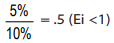since 0 < .5 < 1, wheat is a normal good and fulfils a necessity. b T.V.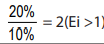Since 2 > 1, T.V. is a luxurious commodity c Bajra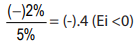Since –.4 < 0, Bajra is an inferior commodity in the eyes of the household d X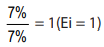Since income elasticity is 1, X has unitary income elasticity e Buttons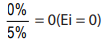Buttons have zero income-elasticity.

It is to be noted that the words ‘luxury’, ‘necessity’, ‘inferior good’ do not signify the strict dictionary meanings here. In economic theory, we distinguish them in the manner shown above. Knowledge of income elasticity of demand is very useful for a business firm in estimating future demand for its products. Knowledge of income elasticity of demand helps firms predict the outcome of a business cycle on its market demand. This enables the firm to carry out appropriate production planning and management.

Cross Elasticity of Demand
Price of Related Goods and Demand: The demand for a particular commodity may change due to changes in the prices of related goods. These related goods may be either complementary goods or substitute goods. This type of relationship is studied under ‘Cross Demand’. Cross demand refers to the quantities of a commodity or service which will be purchased with reference to changes in price, not of that particular commodity, but of other inter-related commodities, other things remaining the same. It may be dened as the quantities of a commodity that consumers buy per unit of time, at dierent prices of a ‘related article’, ‘other things remaining the same’. The assumption ‘other things remaining the same’ means that the income of the consumer and also the price of the commodity in question will remain constant.
Substitute Products
In the case of substitute commodities, the cross demand curve slopes upwards (i.e. positively) showing that more quantities of a commodity, will be demanded whenever there is a rise in the price of a substitute commodity. In figure 9, the quantity demanded of tea is given on the X axis. Y axis represents the price of coffee which is a substitute for tea. When the price of coffee increases, due to the operation of the law of demand, the demand for coffee falls. The consumers will substitute tea in the place of coffee. The price of tea is assumed to be constant. Therefore, whenever there is an increase in the price of one commodity, the demand for the substitute commodity will increase.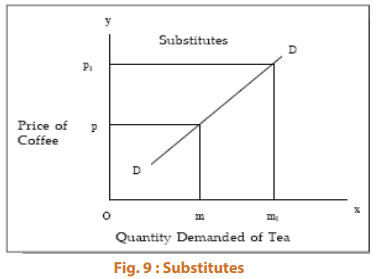Complementary Goods
In the case of complementary goods, as shown in the figure below, a change in the price of a good will have an opposite reaction on the demand for the other commodity which is closely related or complementary. For instance, an increase in demand for pen will necessarily increase the demand for ink. The same is the case with complementary goods such as bread and butter; car and petrol electricity and electrical gadgets etc. Whenever there is a fall in the demand for fountain pens due to a rise in prices of fountain pens, the demand for ink will fall, not because the price of ink has gone up, but because the price of fountain pen has gone up. So, we find that there is an inverse relationship between price of a commodity and the demand for its complementary good (other things remaining the same).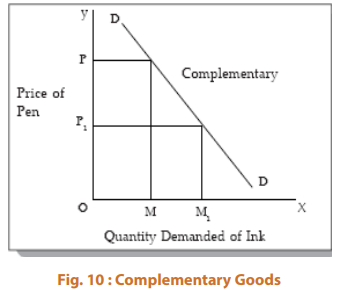A change in the demand for one good in response to a change in the price of another good represents cross elasticity of demand of the former good for the latter good. Here, we consider the effect of changes in relative prices within a market on the pattern of demand.
Symbolically, (mathematically)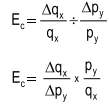Where Ec stands for cross elasticity.
qx stands for original quantity demanded of X.
∆qx stands for change in quantity demanded of X.
py stands for the original price of good Y.
∆py stands for a small change in the price of Y.

If two goods are perfect substitutes for each other, the cross elasticity between them is infinite. Greater the cross elasticity, the closer is the substitute. If two goods are totally unrelated, cross elasticity between them is zero.
If two goods are substitutes (like tea and coffee), the cross elasticity between them is positive, that is, in response to a rise in price of one good, the demand for the other good rises. On the other hand, when two goods are complementary (tea and sugar) to each other, the cross elasticity between them is negative so that a rise in the price of one leads to a fall in the quantity demanded of the other. Higher the negative cross elasticity, higher will be the extent of complementarity.
However, one need not base the classification of goods on the basis of the above definitions. While the goods between which cross elasticity is positive can be called substitutes, the goods between which cross elasticity is negative are not always complementary. This is because negative cross elasticity is also found when the income effect of the price change is very strong. The concept of cross elasticity of demand is useful for a manager while making decisions regarding changing the prices of his products which have substitutes and complements. If cross elasticity to change in the price of substitutes is greater than one, thefirm may lose by increasing the prices and gain by reducing the prices of his products. With proper knowledge of cross elasticity, the firm can plan policies to safeguard against fluctuating prices of substitutes and complements.
Illustration 1:- The price of 1kg of tea is ₹ 30. At this price 5kg of tea is demanded. If the price of coffe rises from ₹ 25 to ₹ 35 per kg, the quantity demanded of tea rises from 5kg to 8kg. Find out the cross price elasticity of tea.
Solution :-
Cross elasticity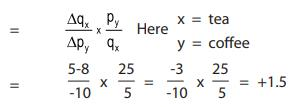The elasticity of demand of tea is +1.5 showing that the demand of tea is highly elastic with respect to coffee. The positive sign shows that tea and coffe are substitute goods.
Illustration 2:- The price of 1 kg of sugar is ₹ 50. At this price 10 kg is demanded. If the price of tea falls from ₹ 30 to ₹ 25 per kg, the consumption of sugar rises from 10 kg to 12 kg. Find out the cross price elasticity and comment on its value.
Solution :-
Cross elasticity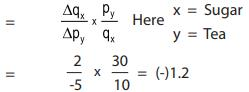Since the elasticity is -1.2 , we can say that sugar and tea are complementary in nature .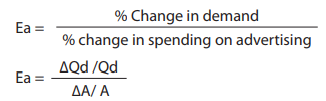Where
∆Qd denotes change in demand.
Qd denotes initial demand.

 Elasticity Interpretation Ea = 0Ea >0 but < 1Ea = 1Ea > 1 Demand does not respond to increase in advertisement expenditureChange in demand is less than proportionate to the change in advertisement expenditure.Demand changes in the same proportion in which advertisement expenditure changes.Demand changes at a higher rate than change in advertisement expenditure.

,

,

,

,

,

,

,

,

,

,

,

,

,

,

,

,

,

,

,

,

,

;